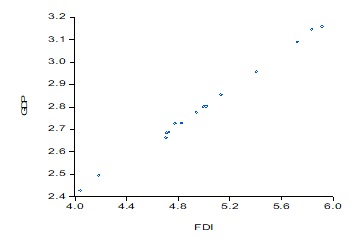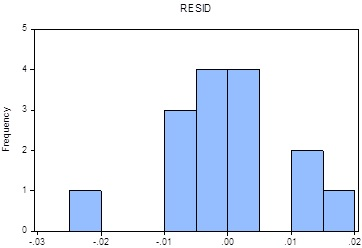```{`Department of Finance and Economics College of Business and Economics Qatar University
Business Statistics II - STAT222 Spring 2020
`}```

Foreign Direct Investment and Economic Growth

Economic theory suggests that foreign direct investment affect the economic growth (the growth of the Gross Domestic Product (GDP)) in developing countries. The objective of this project is to carry out a simple linear regression analysis to examine this theory. Your independent and dependent variables are the growth of the foreign direct investment and the economic growth (the growth of the Gross Domestic Product (GDP)) respectively.

1. State the regression model and determine the least squares regression line.
1. What sign did you expect the estimated parameter to have? Explain.
1. Use the scatter diagram presented in figure 1 to comment on whether it appears that a linear model might be appropriaFigure 1. Scatter diagram

1. Complete the table
 Variable Coefficient Std. Error t-Statistic Prob. C 0.825323 0.025088 …………. 0.0000 FDI 0.395143 ………… 79.13680 0.0000 R-squared 0.997928 Mean dependent var 2.799762 Adjusted R-squared 0.997769 S.D. dependent var 0.215471 S.E. of regression 0.010177 Akaike info criterion -6.213784 Sum squared resid 0.001346 Schwarz criterion -6.119377 Log likelihood 48.60338 Hannan-Quinn criter. -6.214790 F-statistic 6262.633 Durbin-Watson stat 2.011334 Prob(F-statistic) 0.000000

Table 1. Estimation results

1. Write the regression line.
1. Conduct a test of the coefficient of correlation to determine at the 5% significance level whether FDI is related to the GDP, as the theory suggest
1. Conduct a test of the regression slope to determine at the 5% significance level whether a positive and significant linear relationship exists between the two varia
1. Does it appear that the error variable is normally distributed? Ex (Refer to the Histogram of the residuals presented in figure 2.Figure 2. Histogram of the residuals